Page | 1 May 5, 2014
APN-065 Rev A
APN-065:
Determining Rotations for
Inertial Explorer and SPAN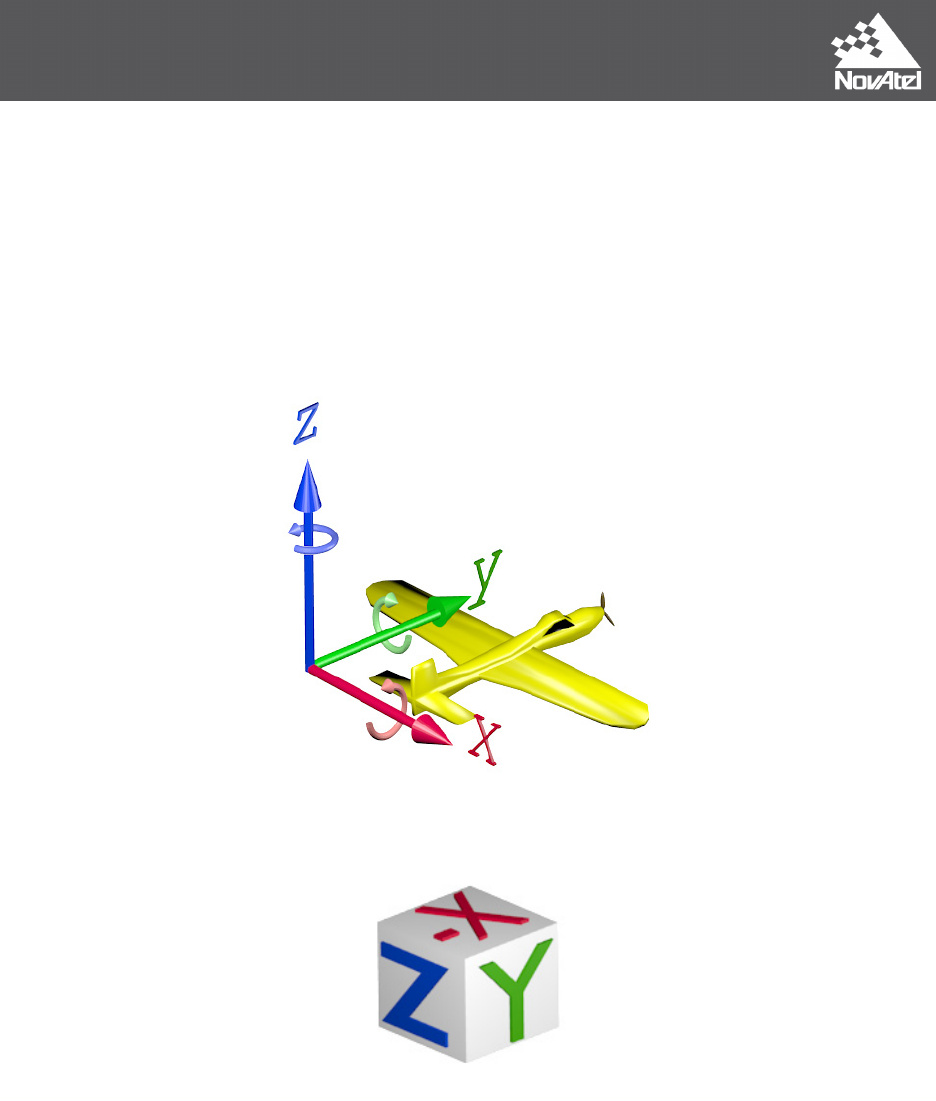Page | 2 May 5, 2014
Both Inertial Explorer (IE) and SPAN use intrinsic ZXY-order Euler angles to define the rotation
between the IMU and output (vehicle) frames, but each has a different approach to how the user
should come up with the input angles. The objective is to determine the rotation from the vehicle
to the target frame (IMU).
Whenever possible, mount the IMU without rotations (Y forward, X right, Z up). This will
help reduce the possibility for errors caused by incorrectly configured rotations.
Basic Frames
Figure 1: Cartesian coordinate system (Vehicle Frame)
Figure 2: IMU Frame (Example)
The IMU frame is the objective of the rotations. The rotations are applied to the vehicle frame in
the order ZXY until the IMU frame is reached.
The angles are all in a right handed direction. Using your right hand, with your thumb in the
direction of the vector, the fingers will curl in the direction of positive rotation.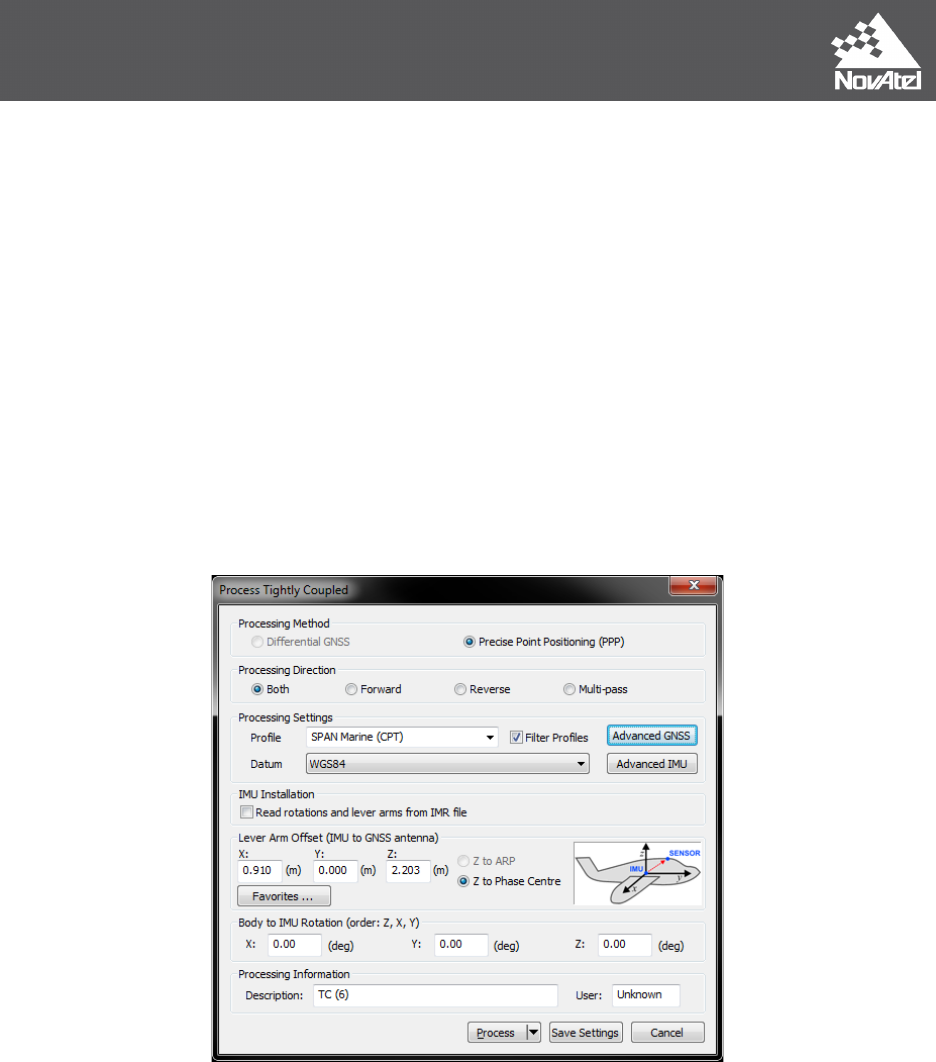Page | 3 May 5, 2014
Inertial Explorer
Rotate around the vehicle Z axis until the vehicle Y axis is in the plane that the IMU Y axis
would sweep out if rotated about its X axis (this is your Z input angle).
Z angle ________
Now rotate around the vehicle X axis until the Y axes match (this is your X input angle).
X angle ________
Now rotate around the vehicle Y axis until both the X and Z axes match (this is your Y input
angle).
Y angle ________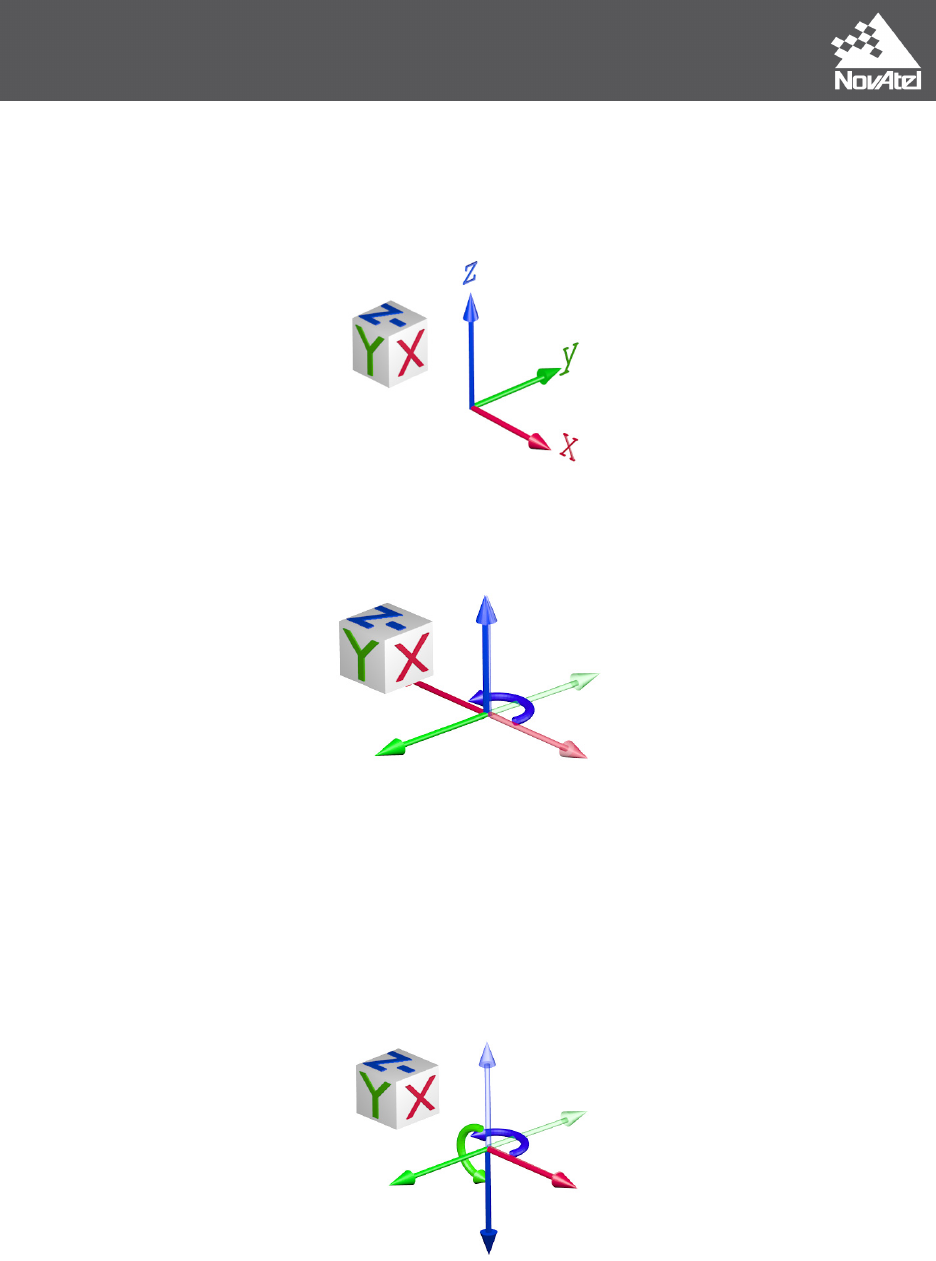Page | 4 May 5, 2014
Example 1 (Inertial Explorer): X = 0 Y = 180 Z = 180
In this example, the IMU (target frame) is mounted with its Y axis backwards, X axis to the right
and Z axis down. The vehicle frame is Y axis forward, X axis right and Z axis up.
Rotate about Z: Starting from the vehicle frame, rotate first about the Z axis (solid blue)
Z = 180 degrees
Rotate about X: Because the Y axis now matches the IMU there is no need to rotate about X
(solid red)
X = 0 degrees
Rotate about Y: Now rotate about the Y axis (solid green) until X and Z match the IMU axes
Y = 180 degrees
Total rotation for input into IE:
X = 0 Y = 180 Z = 180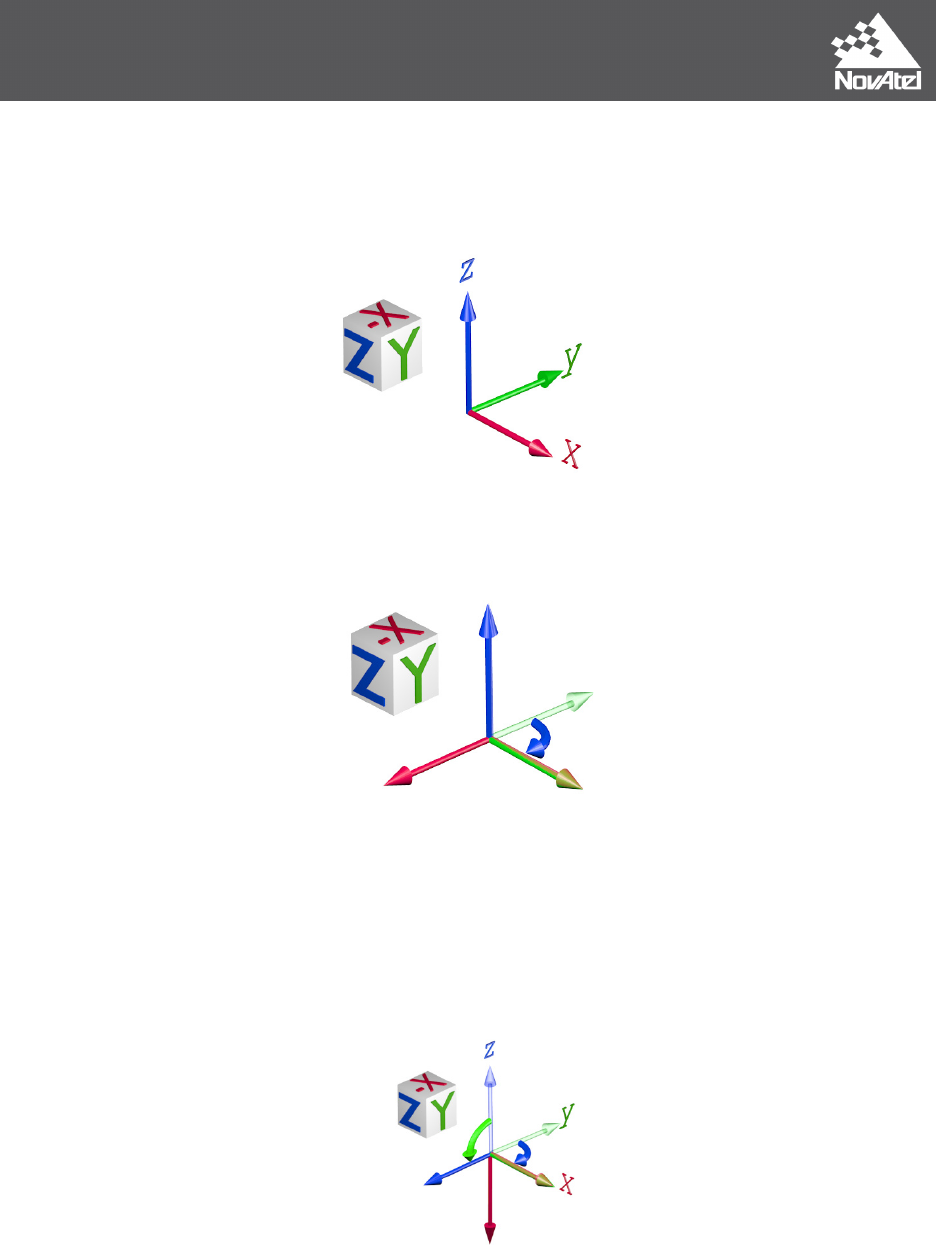Page | 5 May 5, 2014
Example 2 (Inertial Explorer): X = 0 Y = 90 Z = -90
In this example, the IMU (target frame) is mounted with its Z axis backwards, Y axis to the right
and X axis down. The vehicle frame is Y axis forward, X axis right and Z axis up.
Rotate about Z: Starting from the vehicle frame, rotate first about the Z axis (solid blue)
Z = -90 (or 270) degrees
Rotate about X: Because the Y axis now matches the IMU there is no need to rotate about X
(solid red)
X = 0 degrees
Rotate about Y: Now rotate about the Y axis (solid green) until X and Z match the IMU axes
Y = 90 degrees
Total rotation for input into IE:
X = 0 Y = 90 Z = -90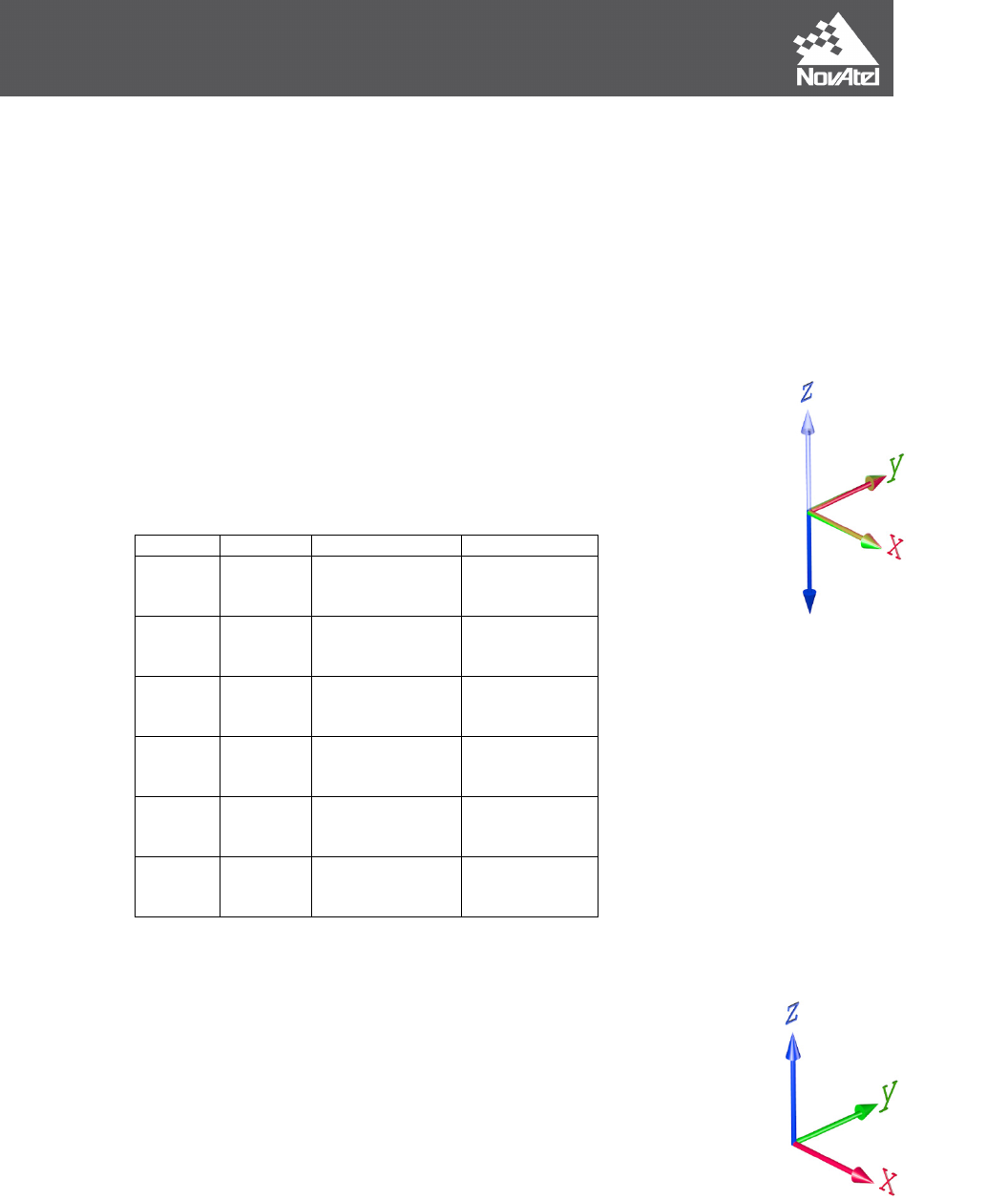Page | 6 May 5, 2014
SPAN
When logging from SPAN, for use later in IE, be sure to log the following logs along with the
standard post processing profile. If logged, IE will automatically populate and use the rotations
from the SPAN file.
LOG IMUTOANTOFFSETSB ONCHANGED
LOG VEHICLEBODYROTATIONB ONCHANGED
Determining what the vehicle to IMU rotation should be:
Which way is up? This defines the mapping during a static alignment.
Or with kinematic alignments, which orientation did you set using the
SETIMUORIENTATION command?
IMU Mapping _______
Table 1: Mapping
Up Axis
Mapping
Before Mapping
After Mapping
X 1
X
Y
Z
Z
X
Y
-X 2
X
Y
Z
-Z
Y
X
Y 3
X
Y
Z
Y
Z
X
-Y 4
X
Y
Z
X
-Z
Y
Z 5
X
Y
Z
X
Y
Z
-Z 6
X
Y
Z
Y
X
-Z
Apply the effect of the mapping to the IMU axes, the result is a new set of IMU axes.
Starting with the vehicle axes (Y forward, X right, Z up), rotate around the
vehicle Z axis until the vehicle Y axis is in the plane that the IMU Y axis would
sweep out if rotated about its X axis (this is your Z input angle).
Z angle ________
Now rotate around the vehicle X axis until the Y axes match (this is your X input
angle).
X angle ________
Now rotate around the vehicle Y axis until both the X and Z axes match (this is your Y input
angle).
Y angle ________
Figure 3: Mapping 6 (Light is
Vehicle, Dark is IMU)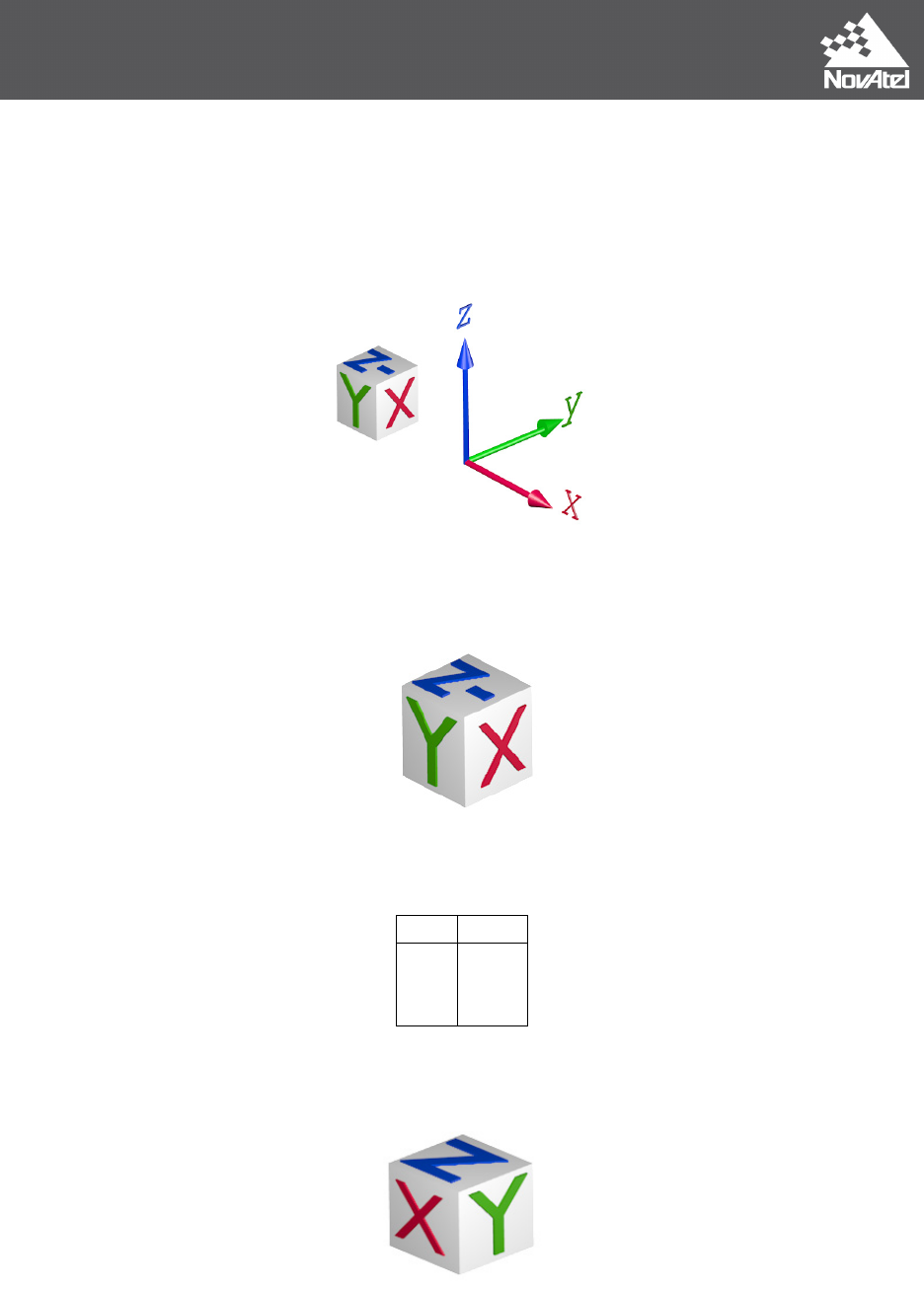Page | 7 May 5, 2014
Example 1 (SPAN):
VEHICLEBODYROTATION 0 0 -90
SETIMUORIENTATION 6
In this example, the IMU is mounted with its Y axis backwards, X axis to the right and Z axis
down. The vehicle frame is Y axis forward, X axis right and Z axis up.
Determine the IMU mapping:
IMU Mapping = 6 (Z down)
Apply the mapping: Using the mapping (6) apply it to the IMU, the result is a new objective
frame. This frame is also called the “computation” frame.
Old
New
X
Y
Z
Y
X
-Z
New IMU Frame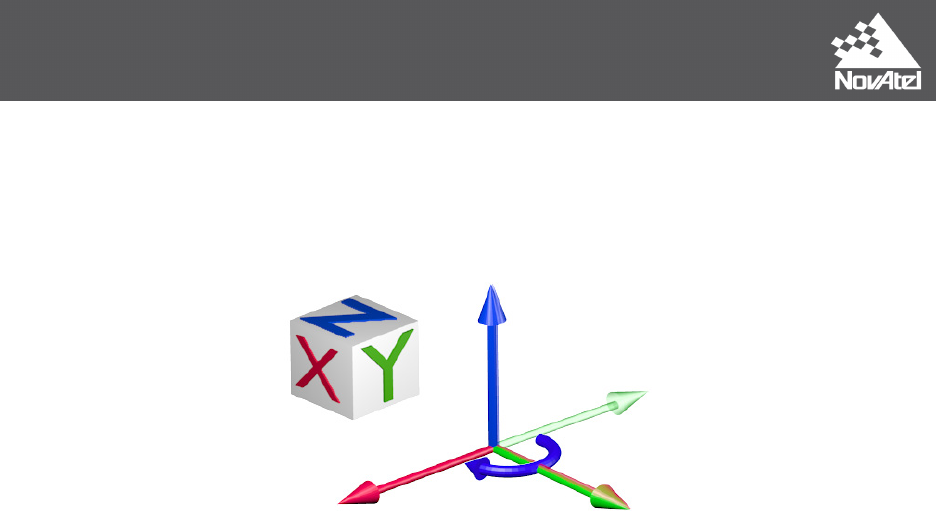Page | 8 May 5, 2014
The objective is to rotate from the vehicle frame to the new objective frame.
Rotate about Z: Starting from the vehicle frame, rotate first about the Z axis (solid blue)
Z = -90 degrees
Rotate about X: Because the Y axis now matches the IMU frame there is no need to rotate